2-Particle Density

## 2-Particle density: Correlated motion of electrons

### Definitions and brief introduction

The basic definitions of the 1- and 2-particle densities for given N-particle state are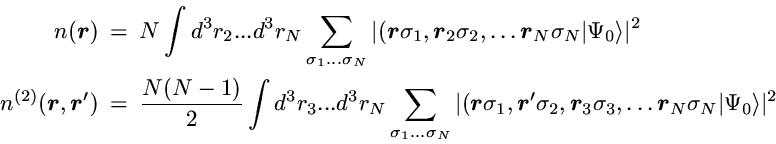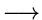The 2-particle density represents the probability to find one particle with arbitrary spin at position r and simultaneously a second particle with arbitrary spin at position r'.

In the case of noninteracting spin-1/2 particles the N-particle state reduces to a Slater determinant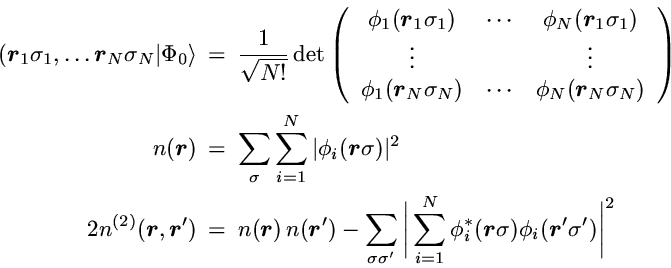The first term in the 2-particle density is the direct product of the probability for finding one particle at point r with the probability to find a particle at r'. If the motion of the particles was completely uncorrelated this product of single-particle probabilities would be the exact result. However, in contrast to classical particles the spin-1/2 fermions are correlated by the Pauli principle, even if a particle-particle interaction is not present. This so-called Pauli correlation is provided by the second term.

For Helium the exact ground state wavefunction has the formDue to the spherical symmetry only 3 spatial coordinates are relevant. One usually chooses the distances r1 and r2 between the electrons and the nucleus (located at the origin) as well as the distance between the two electrons. The 1- and 2-particle densities are then simply given by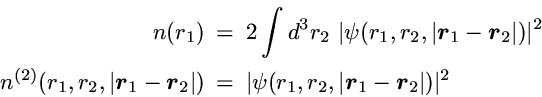Within an effective single-particle description, as the Hartree-Fock or the Kohn-Sham approach, the Helium ground state reduces to a product of single-particle orbitals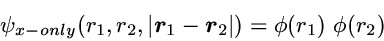While the correlated motion of the two electrons essentially does not show up in the 1-particle density - at least as long as a high quality effective single-particle method is used - it is immediately visible in the 2-particle density, as demonstrated subsequently.

### Plots for Helium

In all plots the position of the nucleus defines the origin. The first electron is kept fixed at different positions on the x-axis (left-right axis), while the position of the second electron is varied. Shown is the probability to find the second electron anywhere in the plane defined by the positions of the three particles. The precise quantity plotted is the radial 2-particle density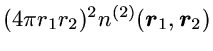which is chosen so that integration over r2 yields the standard radial density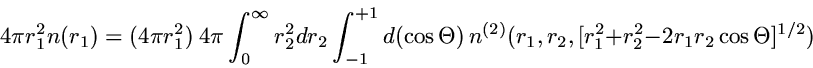For the exact ground state a very accurate variational wavefunction  is utilized (see Appendix). The effective single-particle wavefunction has been obtained via the Optimized Potential Method, using the exact exchange functional of density functional theory. Atomic units are used for all length scales.

#### I) Exact 2-particle density of Helium: r1=(0.559 Bohr, 0, 0) - complete view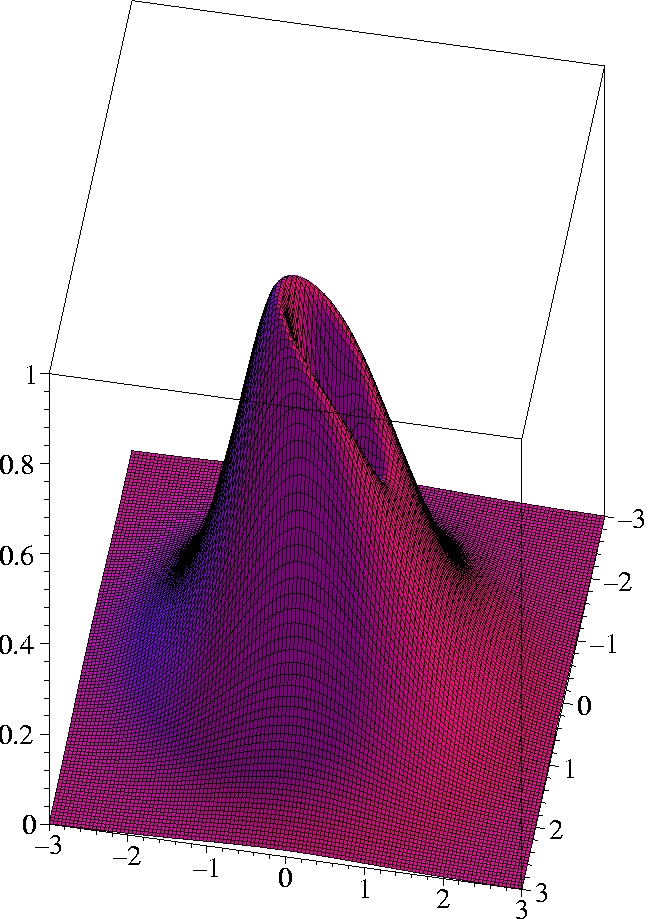x [Bohr]

#### II) Exact 2-particle density of Helium: r1=(0.559 Bohr, 0, 0) - half plane only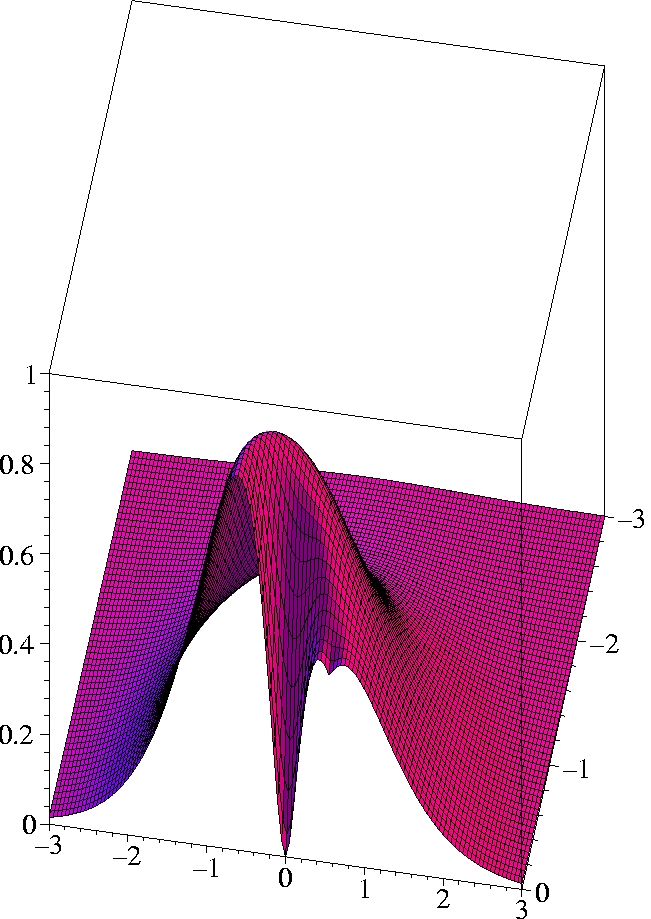x [Bohr]

#### III) Kohn-Sham 2-particle density of Helium: r1=(0.559 Bohr, 0, 0) - half plane only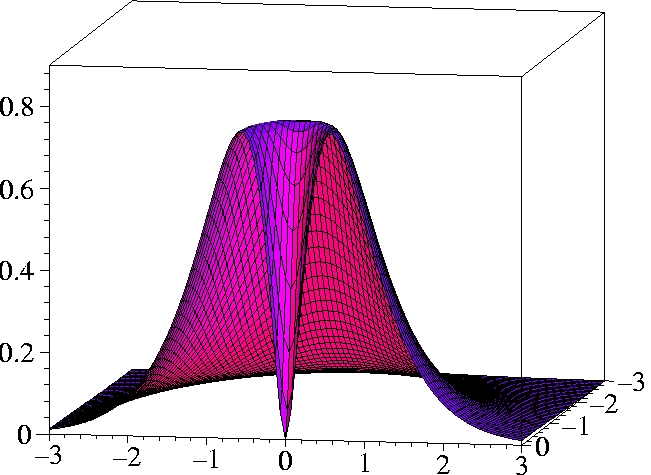x [Bohr]

#### IV) Exact 2-particle density of Helium: r1=(1.0 Bohr, 0, 0)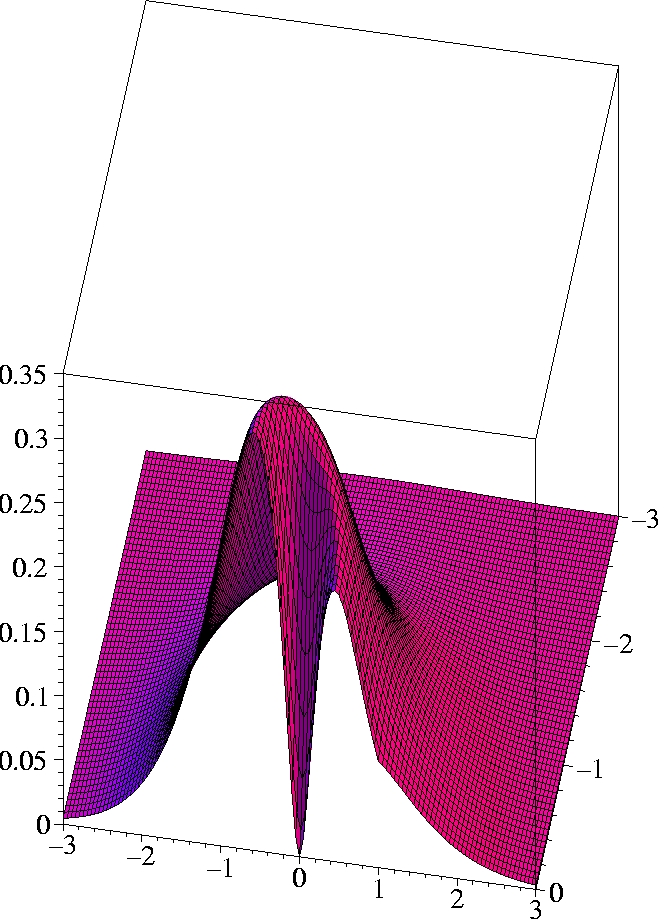x [Bohr]

#### V) Exact 2-particle density of Helium: r1=(2.0 Bohr, 0, 0)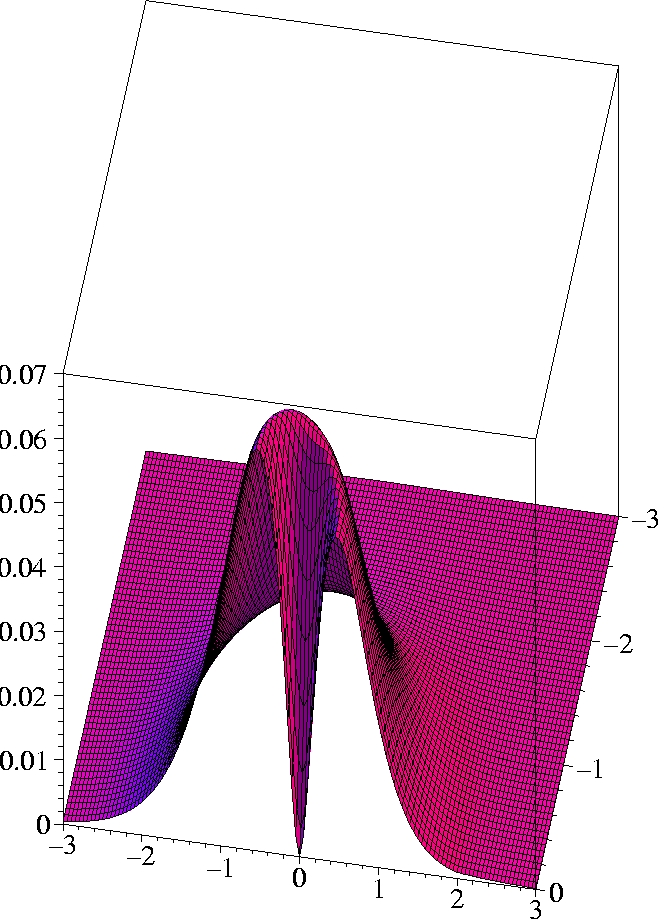x [Bohr]

#### Observations:

• The two electrons preferably move on opposite sides of the nucleus: The likelihood to come close to each other is only half as large as that of remaining on opposite sides (Coulomb correlation).
• The 2-particle density clearly shows the electron-electron cusp at r1= r2, reflecting the short-range Coulomb repulsion.
• While the KS ground state reproduces the exact ground state density exactly, the KS determinant can not reproduce the exact 2-particle density even qualitatively. The motion of the two electrons (Kohn-Sham particles) is uncorrelated.

#### Appendix: Details on variational wavefunction

The correlated wavefunction used for this comparison is the most elaborate variational wavefunction (38 parameters) given in the original work of Kinoshita . It has the form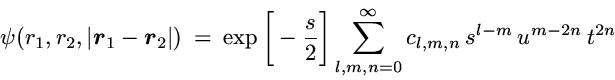with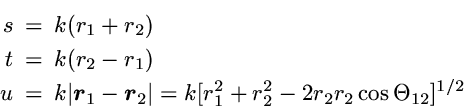and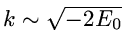,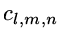= variational parameters

#### References

1. T. Kinoshita, Phys. Rev. 105, 1490 (1957).

Home Top